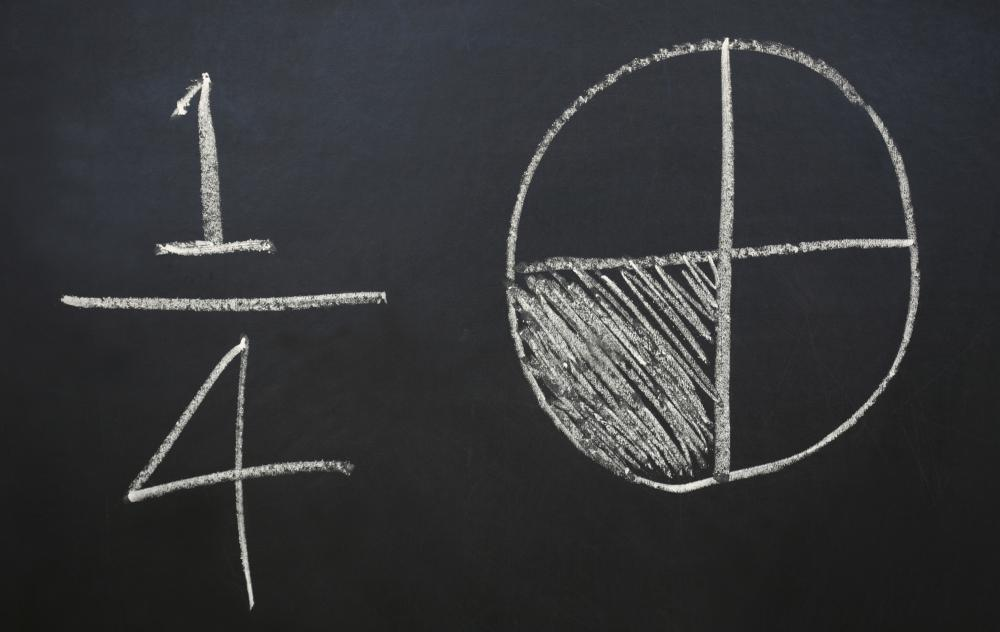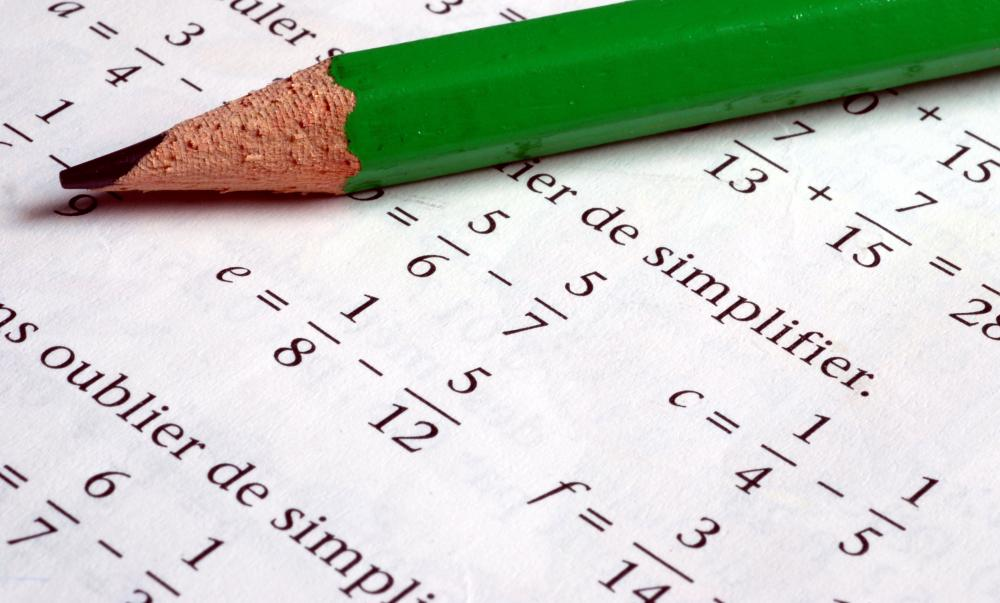# What is a Numerator?Mary McMahon

A numerator is the top part of a fraction, a mathematical expression which expresses part of a whole. For example, 7/19 is a fraction, with the numerator of that particular fraction being “7.” Likewise, 8/3 is also a fraction. The bottom part of a fraction is known as the denominator, with some people using the term “nominator” to talk about numerators. The numerator describes the number of parts of the whole involved in the fraction.The numerator of a fraction represents the parts of a whole.

Fractions can be written with a vertical or horizontal bar, depending on personal taste and convention. In complex equations, fractions are often written with horizontal bars so that they are easy to see. Conventionally, fractions are simplified into what are known as irreducible fractions, so it would be unusual see a fraction like 3/9, which would be represented instead as 1/3. The ability to simplify fractions is also important, as it allows people to see the relation between various fractions, and to do equations with fractions. For example, the connection between 8/12 and 3/9 is much easier to see when these fractions are simplified to 2/3 and 1/3.In a fraction, the numerator is the top number written above the dividing line.

When people simplify fractions to compare them, they start by looking for the lowest common denominator, the smallest multiple of the denominators involved in the fractions being compared. In the example above, the lowest common denominator is 36, because both 12 and 9 can be multiplied to create 36, 12 three times and nine four times. This example is fairly easy to calculate; other fractions can make it much harder to find lowest common denominators.

By multiplying the numerator and denominator in the first fraction by three and in the second fraction by four to reach the lowest common denominator while retaining the correct proportions in the fraction, the fractions could be expressed as 24/36 and 12/36, respectively. These fractions are very clunky, so the next step involves looking for the greatest common divisor, the biggest number which can be used to divide the numerators and denominators while keeping them as whole numbers.

The greatest common divisor in our example happens to be 12. When the numerators and denominators are all divided by 12, the resulting fractions are 2/3 and 1/3. It is important to retain the relationship between the numerator and the denominator, to ensure that the fraction stays the same, which means that any operation performed on a numerator needs to be performed on a denominator, and vice versa. In our example, if someone failed to multiply the numerator of 8/12 when multiplying the denominator, the resulting fraction would be 8/36, a very different fraction from 24/36.Mary McMahon

Ever since she began contributing to the site several years ago, Mary has embraced the exciting challenge of being a wiseGEEK researcher and writer. Mary has a liberal arts degree from Goddard College and spends her free time reading, cooking, and exploring the great outdoors.

## You might also Likeaishia

@gimbell - Rationalizing numerators isn't so tough once you get a few formulas under your belt. It's also important to know if you need to take any higher math classes, like calculus.

Since I can't type in formulaic symbols here in the comment window, I'm going to do what my calculator's buttons do and type "sqrt()" for the square root of the number inside the parentheses. To show when something is squared, I'll put "^2".

Here we go.

To rationalize a numerator (or a denominator, actually) that contains an expression such as the following:

sqrt(a) - (sqrt(b)

...then you would multiply both the numerator and the denominator by what is called a conjugate radical -- the conjugate radical for the above expression looks like this:

sqrt(a) + (sqrt(b)

After you multiply the numerator and the denominator by the conjugal radical, you use the formula for finding the difference of a square on the results and you're done. Not too tough, right?

The formula for finding the difference of a square is as follows:

(sqrt(a) - sqrt(b)) (sqrt(a) + sqrt(b)) = sqrt(a)^2 - sqrt(b)^2 = a - b

Hope this helps!

gimbell

Math gives me so much trouble! When I first started learning fractions, I had a lot of trouble remembering which was the numerator and which was the denominator. The names are such a mouthful, it made it hard to repeat them to ask anybody else, either.

By now I've figured out what a numerator is, but now I get confused about how to rationalize the numerator whenever I do fraction problems. To me, nothing about math is rational -- I don't know how people come up with the numbers they end up with when they do the same problems I've been struggling with.

I hope that there is some easy to remember trick to rationalizing numerators somewhere, or I'm doomed when test time rolls around! Any help anybody can offer would be great.